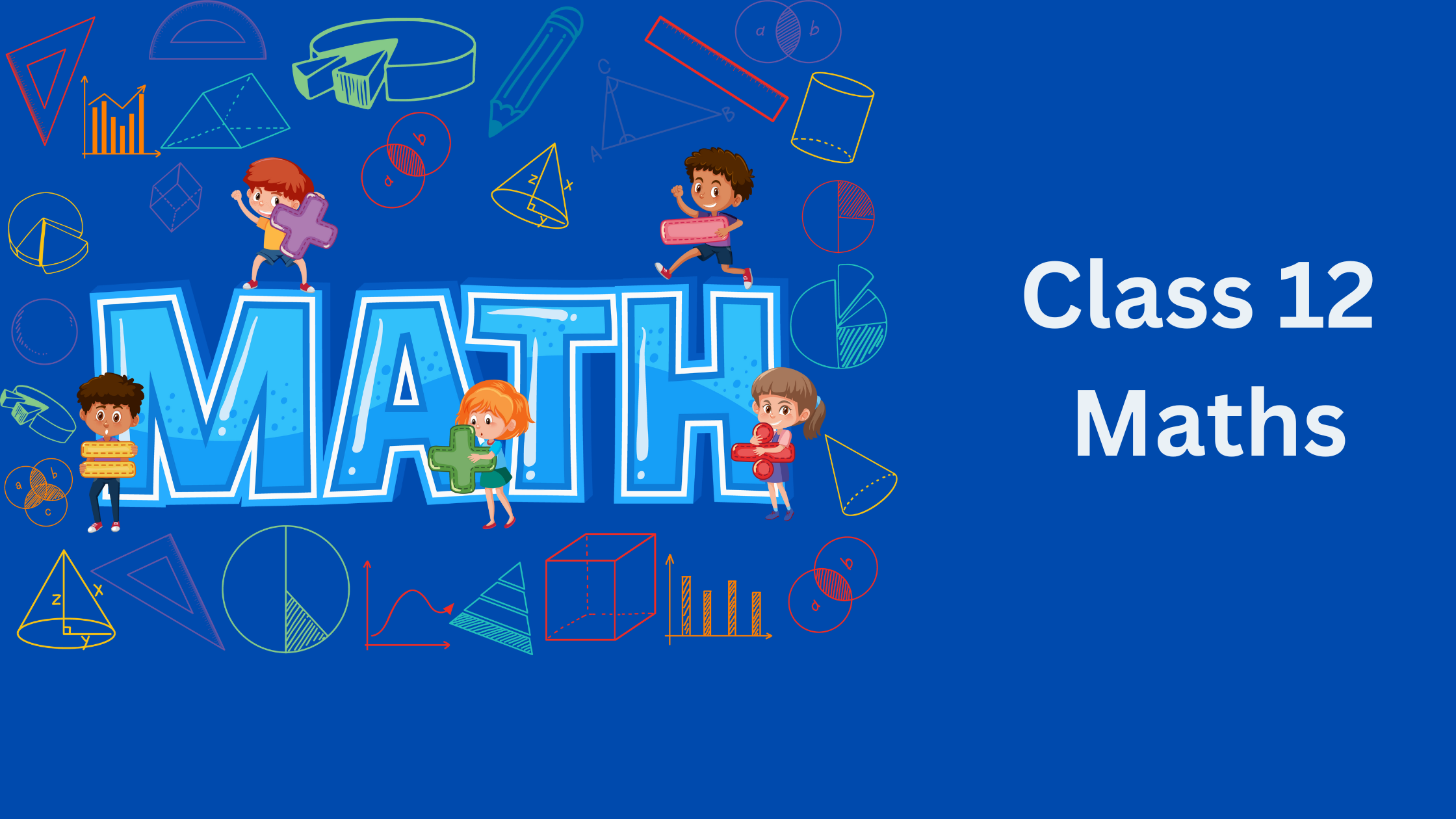# Class 12 Maths NCERT Solutions and Notes

•• Master Class 12 Maths And Be Successful in exams.Here find Class 12 Maths Notes, assignments,NCERT Solutions concept maps and lots of study material for easy learning and understanding. We have lots of study material written in easy language that is easy to follow.

1. First read and understand the notes.
2. Try to go through the solved examples of of the chapter you are reading
3. Now it is the time to solve unsolved assignments and worksheets. You will find plenty of them here or on the respective chapter page.
All the best for better learning of your maths concepts. Hope we helped you when you were looking for class 12 Maths notes .

## Introduction

Mathematics is the study of numbers, equations, functions, and geometric shapes and their relationships.It in used all the "hard" sciences, such as biology, chemistry, and physics; the "soft" sciences, such as economics, psychology, and sociology; engineering fields, such as civil, mechanical, and industrial engineering; and technological fields such as computers, rockets, and communications.So it is important for the basics to be very clear from the starting .We are creating the material so that students can understand and develop clear understanding of Mathematics. Here are we are giving the Class 12 Maths study material,Class 12 Maths worksheets ,NCERT solutions for Class 12 maths,exam paper, Maths problem and solution to help student practice maths . This will help in getting good marks in examination.

## Chapter -1 Relations and Functions

Notes
NCERT Solutions & worksheets

## Chapter -2 Inverse Trigonometric Functions

Notes
NCERT Solutions & worksheets

Notes
NCERT solutions
Worksheets

Notes
Worksheets

Notes
NCERT solutions

NCERT solutions

NCERT solutions

NCERT solutions

Notes
Assignments

Notes
NCERT solutions

## Class 12 Board Test paper

Previous year(2023/2016/2015/2014) CBSE paper
CBSE Sample Paper(2023-2024)

## Frequently asked Questions on CBSE Class 12 Maths

### How many chapters are there in Class 12 Maths?

There are total 13 chapters in Class 12 Maths as per the rationalized Syllabus. All the chapters are required for assessment

### What is the best books for Class 12 Maths?

Couple of book
(a)NCERT Class 12 Maths textbook
(b)NCERT Class 12 Exemplar
(c)R D Sharma

### Which is the easiest chapter of class 12 Maths NCERT Text book?

Matrices and Determinants are the easiest chapter

### Is Class 12 Maths NCERT Text book Sufficient for CBSE Exams?

It is sufficient if you go through all the questions in the NCERT text book thoroughly and practice them all.However to enhance your maths skill , you can go for NCERT exemplar and other books

### what is the best way to learn the concept in Class 12 Maths?

First go through all the Notes and solved examples and Then do the exercises. Practice Lot of questions. The simple Mantra is Practice .Practice and Practice

### what is the deleted syllabus in Class 12 Maths?

Chapter 1: Relations and Functions
Composite functions, inverse of a function
Chapter 2: Inverse Trigonometric Functions
Elementary properties of inverse trigonometric functions
Chapter 3: Matrices
Concept of elementary row and column operations.
Chapter 4: Determinants
Properties of determinants
Chapter 5: Continuity and Differentiability
Derivative of composite function,Rolle’s and Lagrange's Mean Value,Theorems (without proof) and their geometric interpretation
Chapter 6: Applications of Derivatives
Tangents and normals,Use of derivatives in approximation
Chapter 7: Integrals
Definite integrals as a limit of a sum,
Chapter 8: Applications of the Integrals
Area between any of the two above said curves
Chapter 10: Vectors
Scalar triple product of vectors
Chapter 11: Three dimensional Goemetry
Co-planar lines,Cartesian and vector,Equation of a plane.Angle between (i) two lines, (ii) two planes, (iii) a line and a plane. Distance of a point from a plane
Chapter 12: Linear Programming
Different types of linear programming (L.P.) problems mathematical formulation of L.P. problems
Chapter 13: Probability
Variance of random variable